# 100 200 100 200 300 400 300 400

• Slides: 72\$100 \$200 \$100 \$200 \$300 \$400 \$300 \$400 \$500 \$500Arc Length & Sector Areas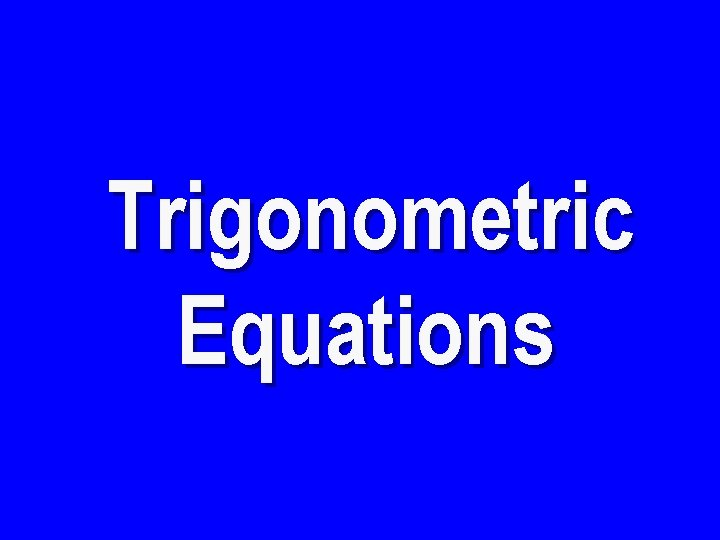Trigonometric Equations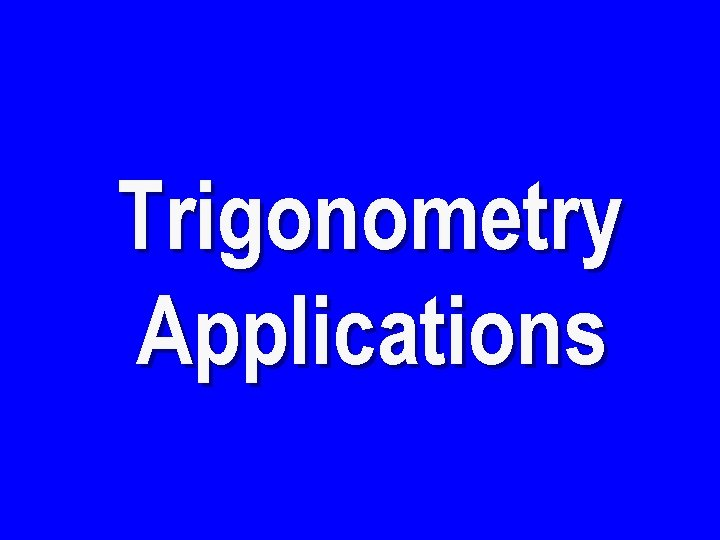Trigonometry Applications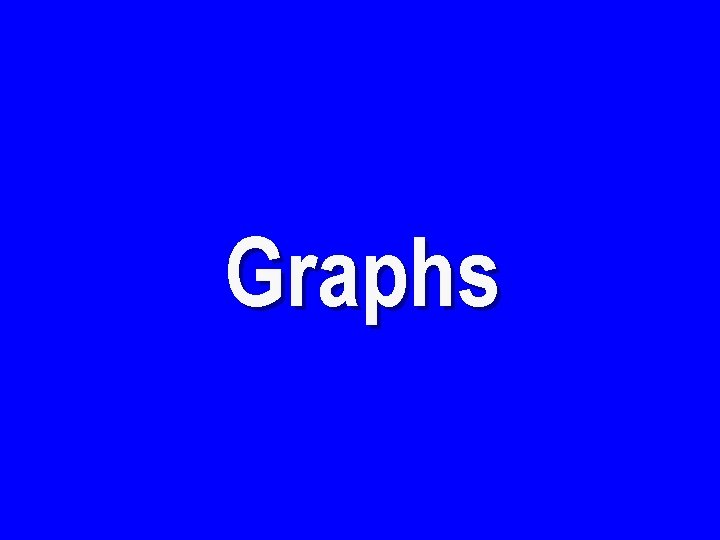Graphs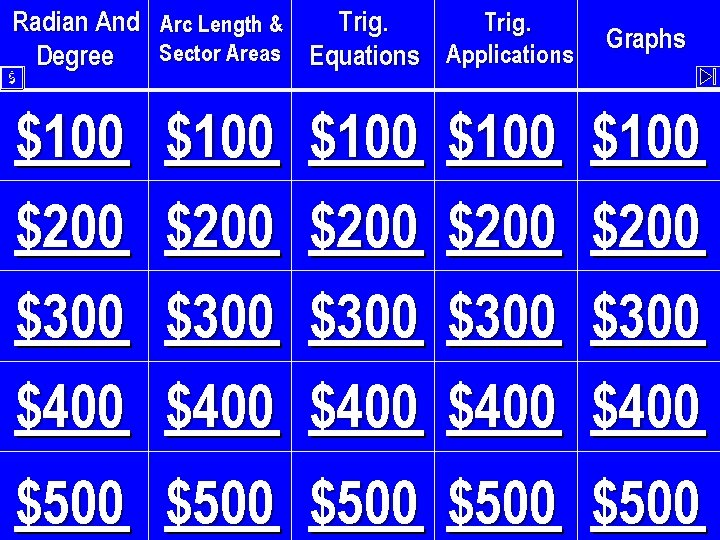Radian And Arc Length & Trig. Sector Areas Equations Applications Degree Graphs \$ \$100 \$200 \$300 \$400 \$100 \$200 \$300 \$400 \$500 \$500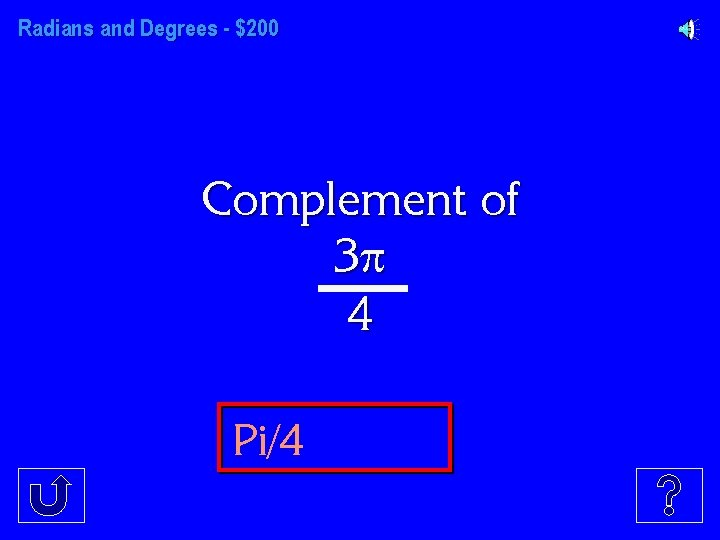Radians and Degrees - \$200 Complement of 3 4 Pi/4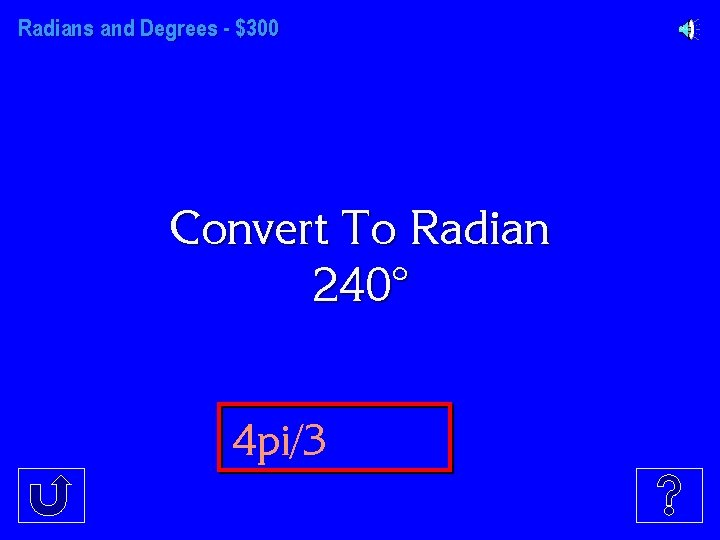Radians and Degrees - \$400 32 M 7 M 64 pi/39 The Radian Measure of Shaded Area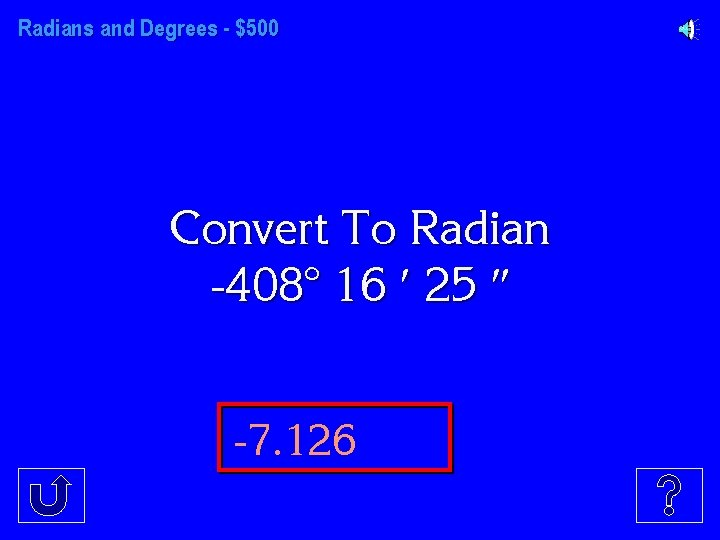Radians and Degrees - \$500 Convert To Radian -408° 16 25 -7. 126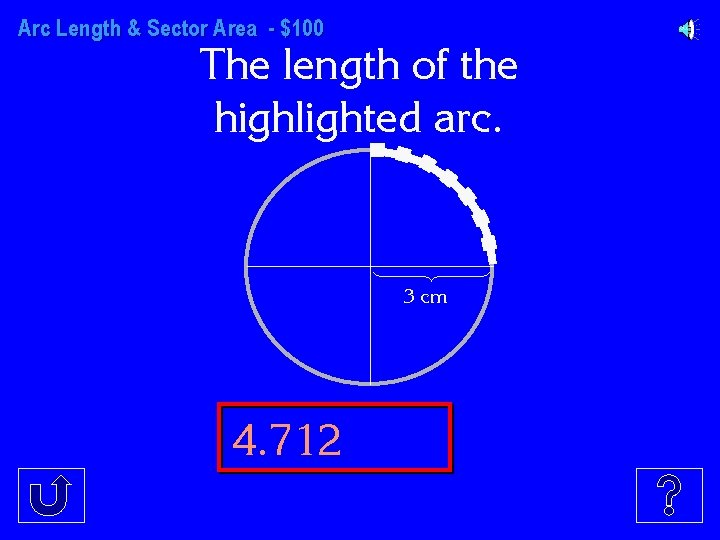Arc Length & Sector Area - \$100 The length of the highlighted arc. 3 cm 4. 712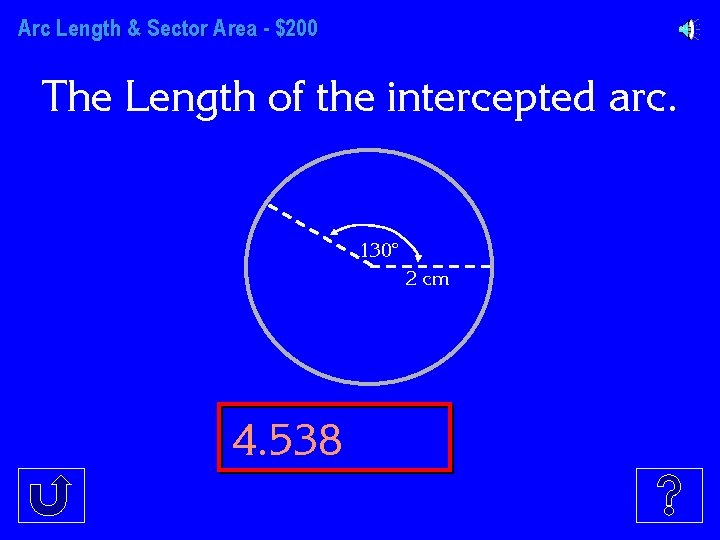Arc Length & Sector Area - \$200 The Length of the intercepted arc. 130° 2 cm 4. 538Arc Length & Sector Area - \$300 The Sector Area of the intercepted arc. 7 _ 6 4 cm 29. 32 cm^2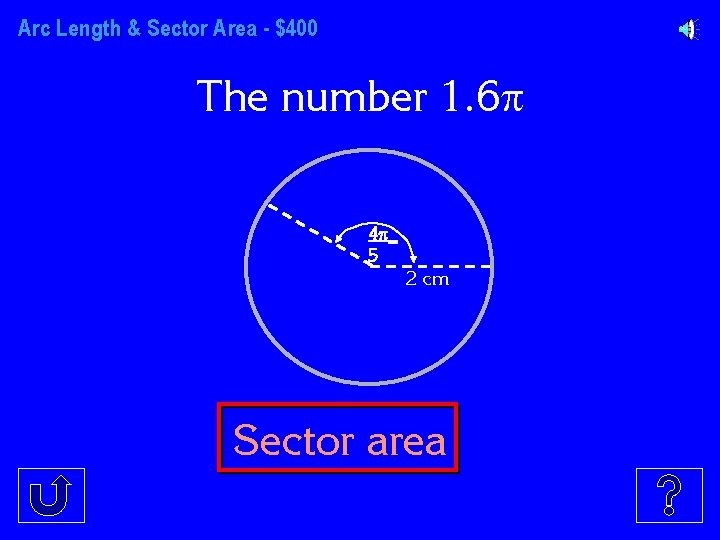Arc Length & Sector Area - \$400 The number 1. 6 4 _ 5 2 cm Sector area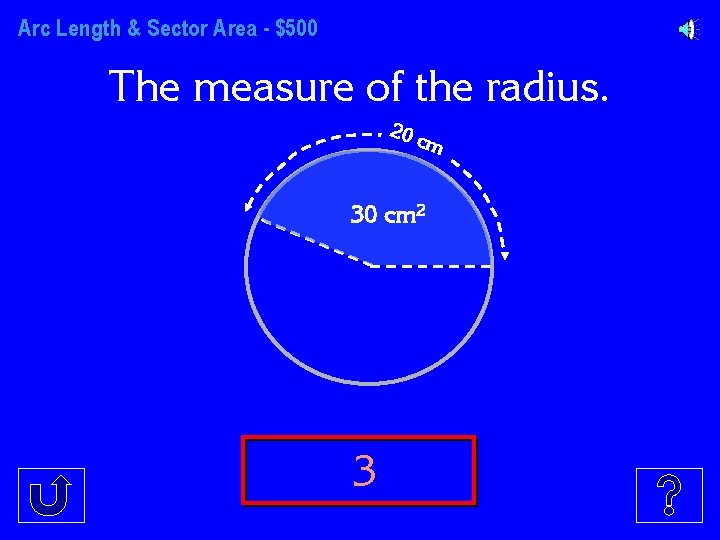Arc Length & Sector Area - \$500 The measure of the radius. 20 c m 30 cm 2 3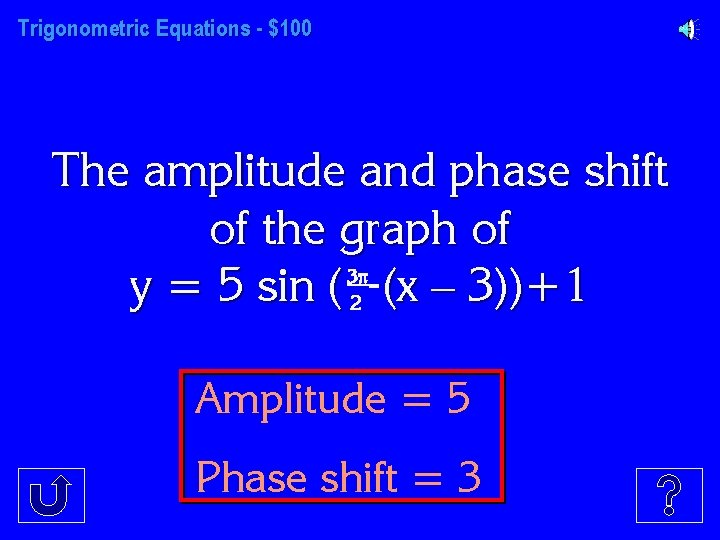Trigonometric Equations - \$100 The amplitude and phase shift of the graph of 3 _ y = 5 sin ( 2 (x – 3))+1 Amplitude = 5 Phase shift = 3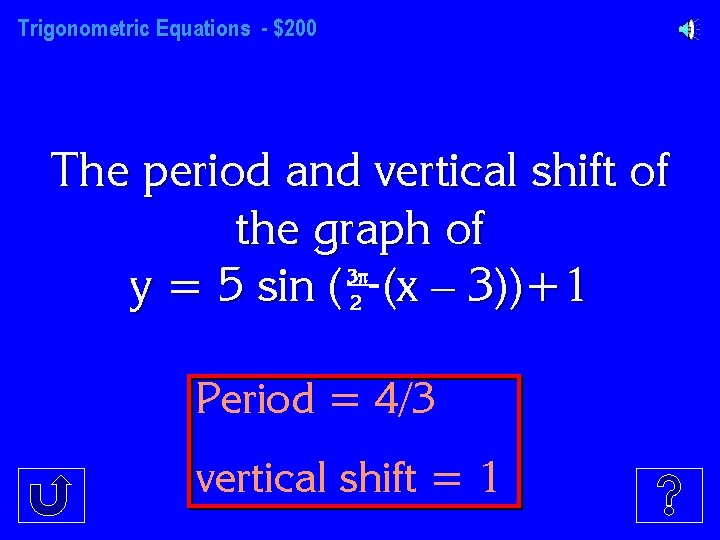Trigonometric Equations - \$200 The period and vertical shift of the graph of 3 _ y = 5 sin ( 2 (x – 3))+1 Period = 4/3 vertical shift = 1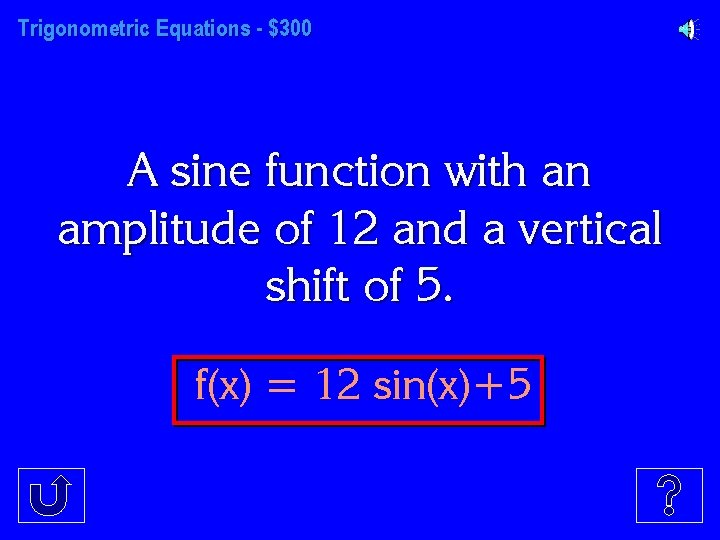Trigonometric Equations - \$300 A sine function with an amplitude of 12 and a vertical shift of 5. f(x) = 12 sin(x)+5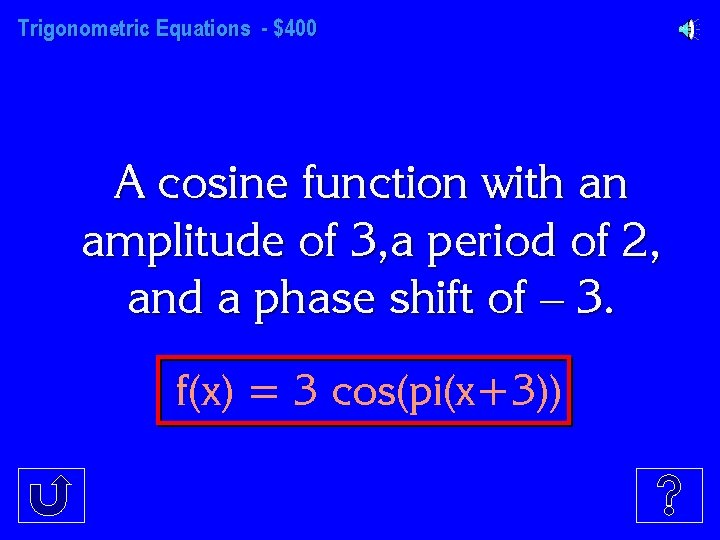Trigonometric Equations - \$400 A cosine function with an amplitude of 3, a period of 2, and a phase shift of – 3. f(x) = 3 cos(pi(x+3))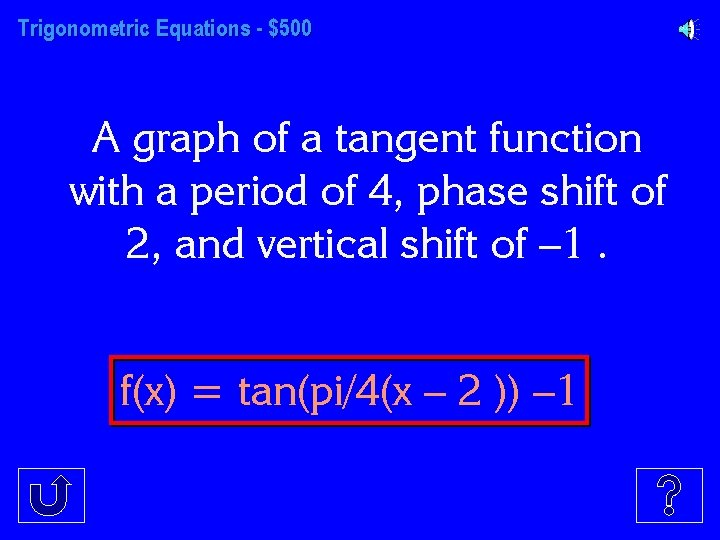Trigonometric Equations - \$500 A graph of a tangent function with a period of 4, phase shift of 2, and vertical shift of – 1. f(x) = tan(pi/4(x – 2 )) – 1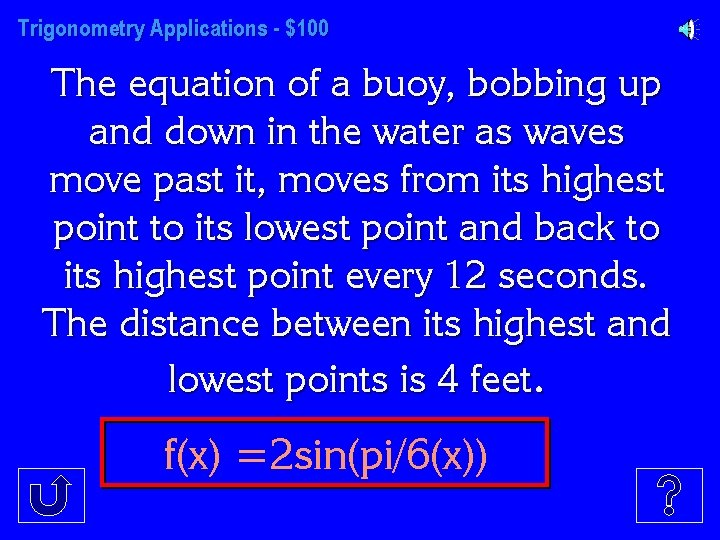Trigonometry Applications - \$100 The equation of a buoy, bobbing up and down in the water as waves move past it, moves from its highest point to its lowest point and back to its highest point every 12 seconds. The distance between its highest and lowest points is 4 feet. f(x) =2 sin(pi/6(x))Trigonometry Applications - \$200 The frequency of a tuning fork that is represented by the graph below. 556 Hz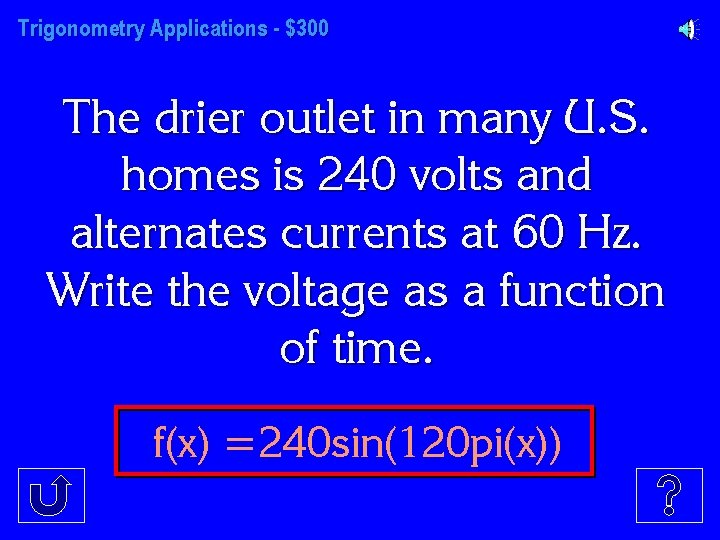Trigonometry Applications - \$300 The drier outlet in many U. S. homes is 240 volts and alternates currents at 60 Hz. Write the voltage as a function of time. f(x) =240 sin(120 pi(x))Trigonometry Applications - \$400 A mathematical function that describes a middle “C” tuning fork. (A middle “C” note has a frequency of 256 Hz. ) f(x) =sin(512 pi(x))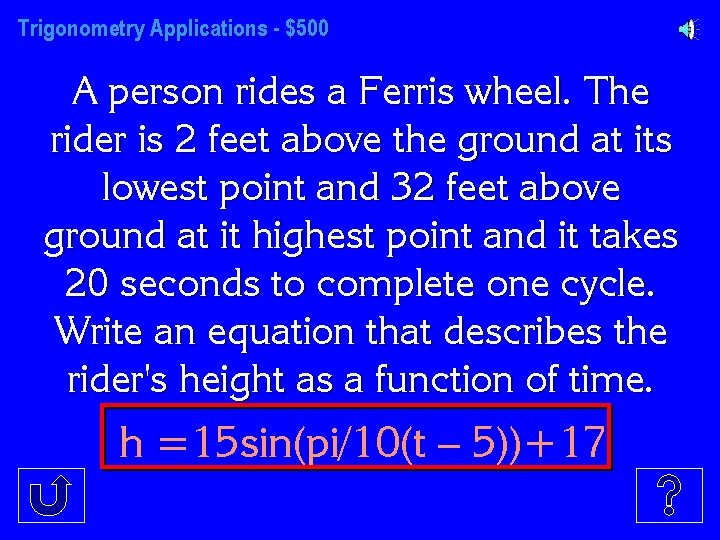Trigonometry Applications - \$500 A person rides a Ferris wheel. The rider is 2 feet above the ground at its lowest point and 32 feet above ground at it highest point and it takes 20 seconds to complete one cycle. Write an equation that describes the rider's height as a function of time. h =15 sin(pi/10(t – 5))+17Graphs - \$100 Domain and Range of y = sin (x) Domain {x|(- , )} Range {y|(-1, 1)}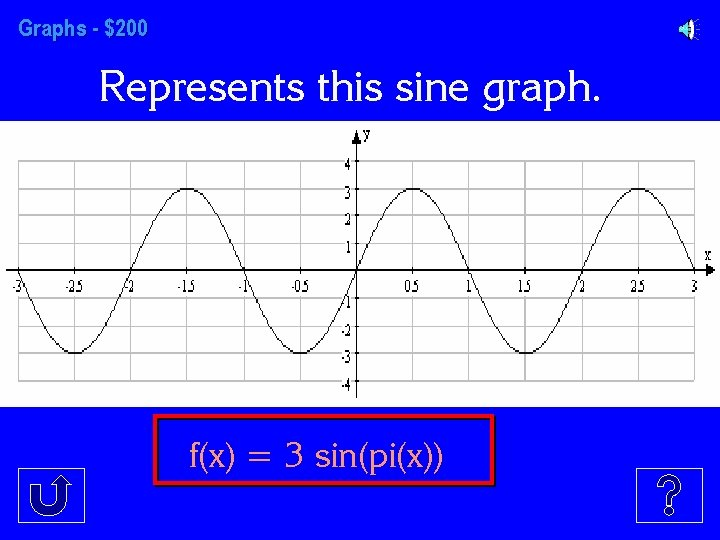Graphs - \$200 Represents this sine graph. f(x) = 3 sin(pi(x))Graphs - \$300 The sketch of a graph of, y=cos (2 x)+3 using increments of 2 for the x -axis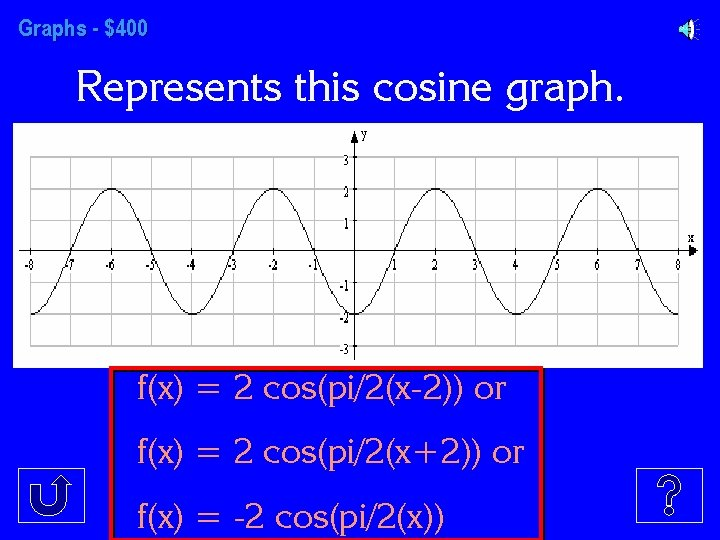Graphs - \$400 Represents this cosine graph. f(x) = 2 cos(pi/2(x-2)) or f(x) = 2 cos(pi/2(x+2)) or f(x) = -2 cos(pi/2(x))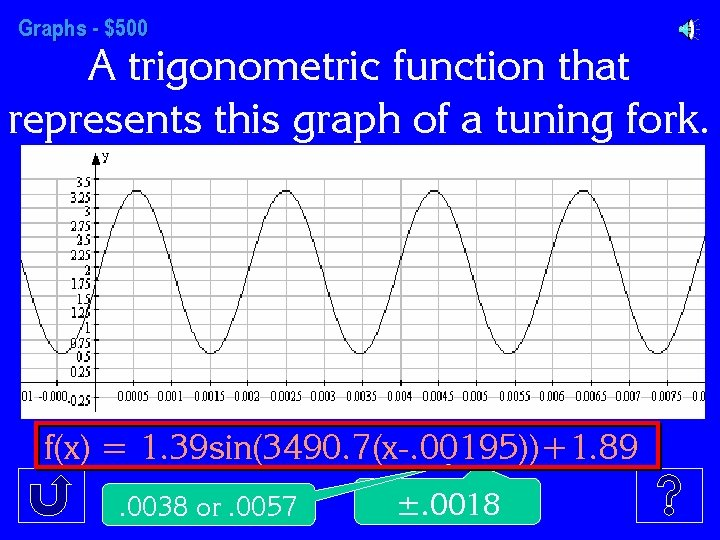Graphs - \$500 A trigonometric function that represents this graph of a tuning fork. f(x) = 1. 39 sin(3490. 7(x-. 00195))+1. 89. 0038 or. 0057 ±. 0018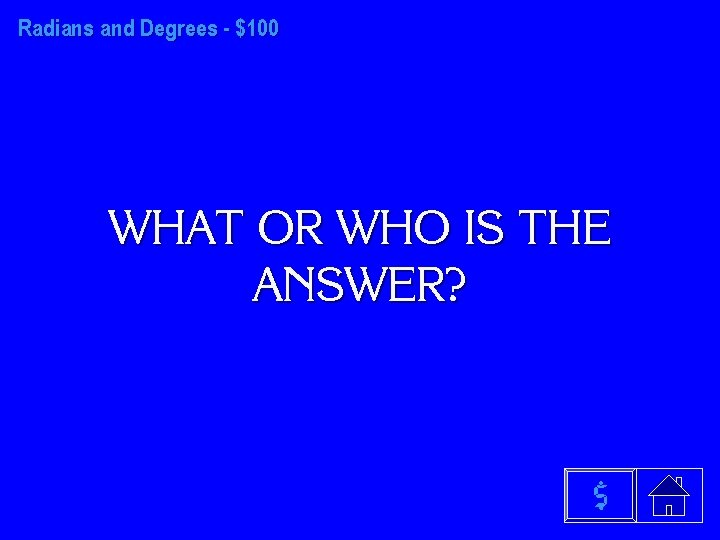Radians and Degrees - \$100 WHAT OR WHO IS THE ANSWER? \$Radian and Degrees - \$200 WHAT OR WHO IS THE ANSWER? \$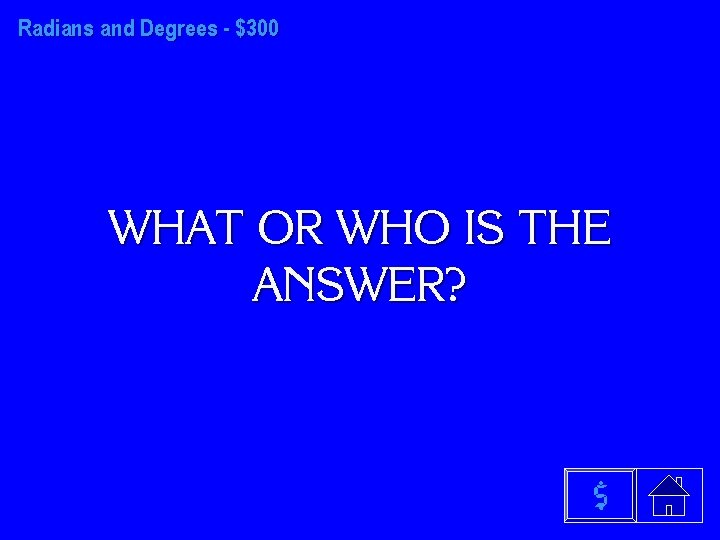Radians and Degrees - \$300 WHAT OR WHO IS THE ANSWER? \$Radians and Degrees - \$400 WHAT OR WHO IS THE ANSWER? \$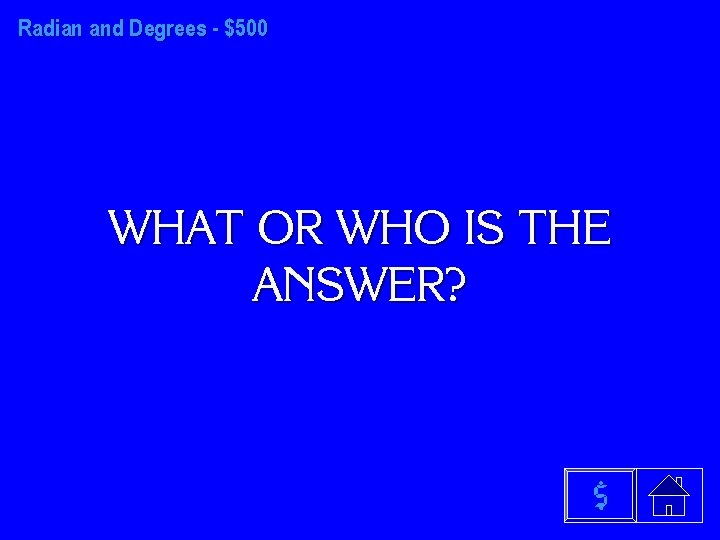Radian and Degrees - \$500 WHAT OR WHO IS THE ANSWER? \$Unit Circle - \$100 WHAT OR WHO IS THE ANSWER? \$Unit Circle - \$200 WHAT OR WHO IS THE ANSWER? \$Unit Circle - \$300 WHAT OR WHO IS THE ANSWER? \$Unit Cirlce - \$400 WHAT OR WHO IS THE ANSWER? \$Unit Circle - \$500 WHAT OR WHO IS THE ANSWER? \$Right Triangles - \$100 WHAT OR WHO IS THE ANSWER? \$Right Triangles - \$200 WHAT OR WHO IS THE ANSWER? \$Right Triangles - \$300 WHAT OR WHO IS THE ANSWER? \$Right Triangles - \$400 WHAT OR WHO IS THE ANSWER? \$Right Triangles - \$500 WHAT OR WHO IS THE ANSWER? \$Trig Functions - \$100 WHAT OR WHO IS THE ANSWER? \$Trig Functions - \$200 WHAT OR WHO IS THE ANSWER? \$Trig Functions - \$300 WHAT OR WHO IS THE ANSWER? \$Trig Functions- \$400 WHAT OR WHO IS THE ANSWER? \$Trig Functions - \$500 WHAT OR WHO IS THE ANSWER? \$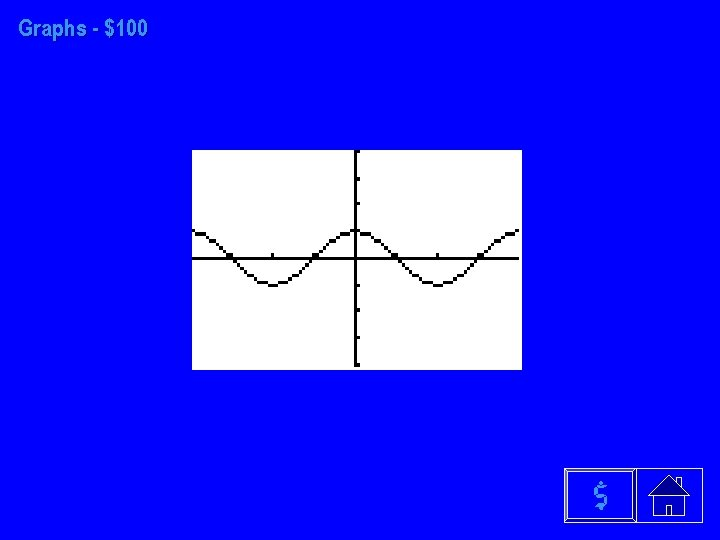Graphs - \$100 \$Graphs - \$200 WHAT OR WHO IS THE ANSWER? \$Graphs - \$300 WHAT OR WHO IS THE ANSWER? \$Graphs - \$400 WHAT OR WHO IS THE ANSWER? \$Graphs - \$500 \$Applications \$FINAL CATEGORY Two Fire Towers are 20 Miles apart, Tower A being Due North of Tower B. A fire is spotted at S 14°E and N 34°E, respectively from each tower. Find the distance of fire from the line segment AB \$FINAL CATEGORY WHAT OR WHO IS THE FINAL ANSWER? \$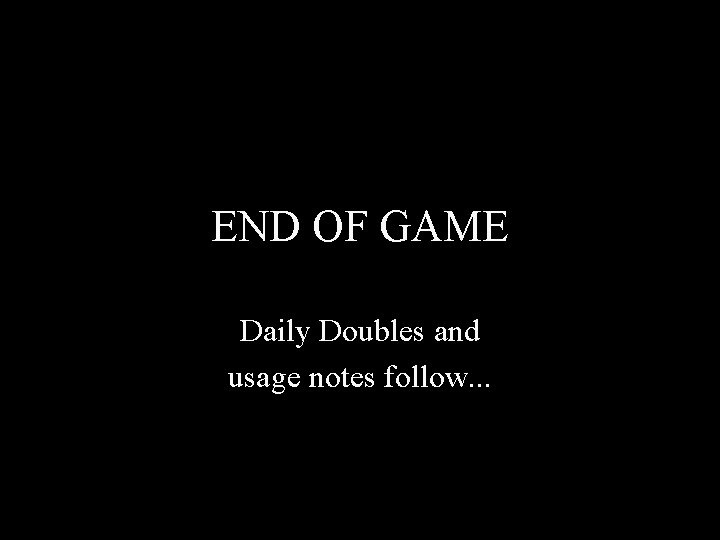END OF GAME Daily Doubles and usage notes follow. . .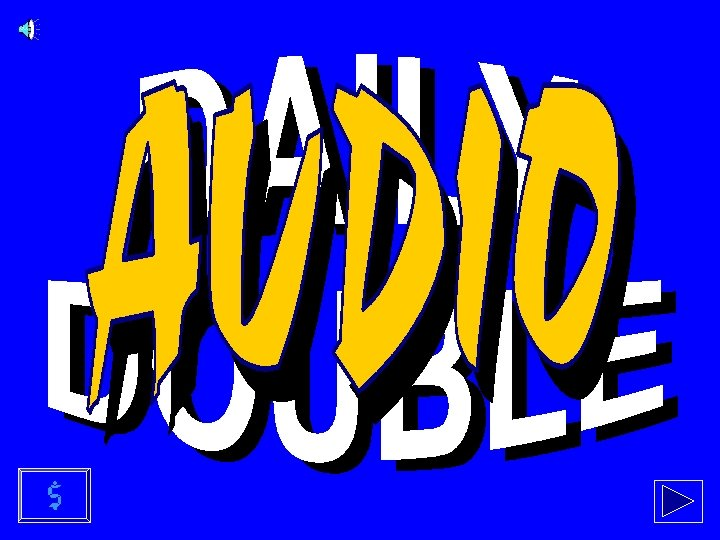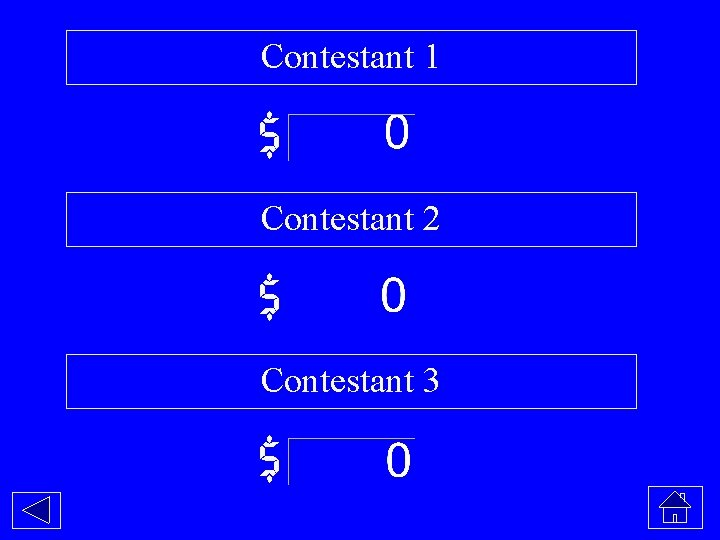Contestant 1 \$ Contestant 2 \$ Contestant 3 \$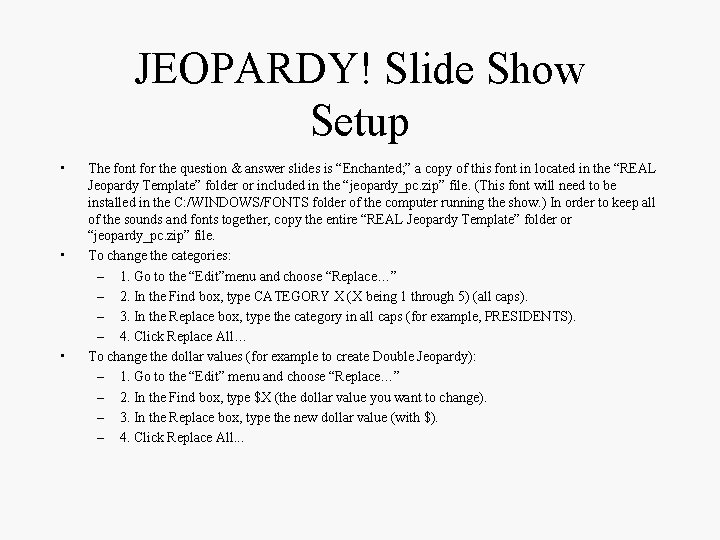JEOPARDY! Slide Show Setup • • • The font for the question & answer slides is “Enchanted; ” a copy of this font in located in the “REAL Jeopardy Template” folder or included in the “jeopardy_pc. zip” file. (This font will need to be installed in the C: /WINDOWS/FONTS folder of the computer running the show. ) In order to keep all of the sounds and fonts together, copy the entire “REAL Jeopardy Template” folder or “jeopardy_pc. zip” file. To change the categories: – 1. Go to the “Edit”menu and choose “Replace…” – 2. In the Find box, type CATEGORY X (X being 1 through 5) (all caps). – 3. In the Replace box, type the category in all caps (for example, PRESIDENTS). – 4. Click Replace All… To change the dollar values (for example to create Double Jeopardy): – 1. Go to the “Edit” menu and choose “Replace…” – 2. In the Find box, type \$X (the dollar value you want to change). – 3. In the Replace box, type the new dollar value (with \$). – 4. Click Replace All. . .